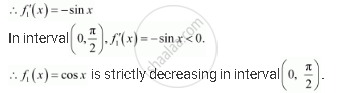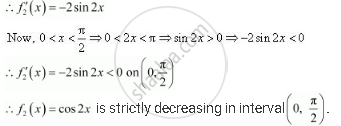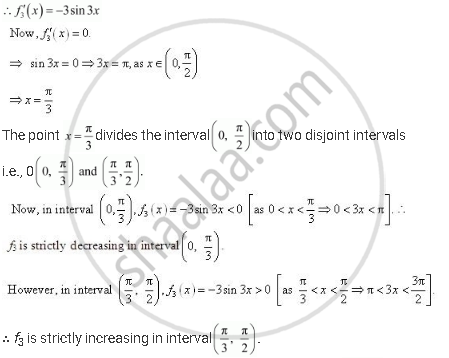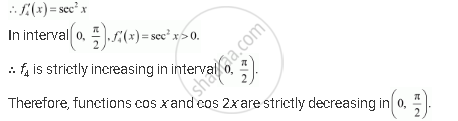Share

# Which of the Following Functions Are Strictly Decreasing on (0, Pi/2)? - CBSE (Commerce) Class 12 - Mathematics

ConceptIncreasing and Decreasing Functions

#### Question

Which of the following functions are strictly decreasing on (0, pi/2)?

(A) cos x

(B) cos 2x

(C) cos 3x

(D) tan x

#### Solution

(A) Let f_1 (x) =  cos x(B) Let f_2(x) = cos 2x(C) Let f_1(x) =  cos 3xHence, f3 is neither increasing nor decreasing in interval (0, pi/2)

Hence, the correct answers are A and B

(D) Let f_4(x) = tan xHence, the correct answers are A and B.

Is there an error in this question or solution?

#### APPEARS IN

NCERT Solution for Mathematics Textbook for Class 12 (2018 to Current)
Chapter 6: Application of Derivatives
Q: 12 | Page no. 206

#### Video TutorialsVIEW ALL 

Solution Which of the Following Functions Are Strictly Decreasing on (0, Pi/2)? Concept: Increasing and Decreasing Functions.
S